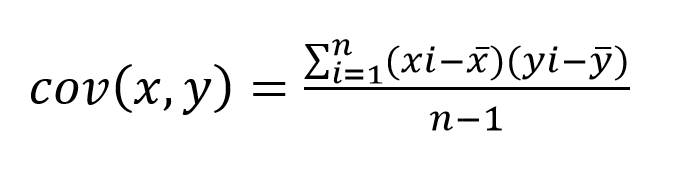# Covariance

Covariance is what it sounds like. It describes how two variables “covary” with each other, given by the equation (sample):where:

• xi and yi denote observations of a variable x, and a variable y, respectively
• n represents the number of observations
• x̄ and ȳ represent the averages of our two variables, respectively

To find Covariance we simply take each observation of both x and y, and subtract it’s mean from it. Then we multiply the results together, repeat this process for all observations and sum all of our results to get the numerator of our equation. We divide this by the number of our observations, less one. For a detailed walk-through in excel, click here.